Welcome

# Documentation Wiki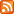All Forum Wiki Wiki & Forum Forum Archive

# Sine Oscillator

## Name

Sine Oscillator: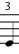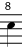sine oscillator

## Description

The Sine Oscillator Agent is EigenD's built-in sine oscillator plug-in.

## Ports

#### Volume input

• Name: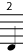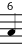volume input

• Input, Set
• Type: Number
• Range: 0 to 1; default = 0

The volume input is normally used to receive volume information from upstream Agents.

#### Frequency input

• Name:frequency input

• Input, Set
• Type: Number
• Range: 0 to 96000; rest = 440

The frequency input is used in the main to receive frequency signals from an upstream Agent (normally from the Scaler's frequency output in this case) to be processed accordingly, but can also be set by the user.

#### Detune input

• Name:detune input

• Input, Set
• Type: Number
• Range: -1200 to 1200

The detune input is normally used to detune the output frequency of the Sine Oscillator using a value (in cents) set by the user.

#### Audio output

• Name: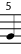audio output

• Output
• Type: Audio

The audio output is used for sending audio data to downstream Agents.

None

None

## Examples

Before using the Belcanto examples below, please make sure you have the desired Sine Oscillator in the conversation using: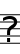sine oscillator X listen

where X is the number of the desired Sine Oscillator.

#### Setting the value of the frequency input

To set the value of the frequency input, usefrequency input to X set

where X is the value of the desired frequency.

#### Setting the value of the detune input

To set the value of the detune input, usedetune input to X set

where X is the value of the desired detune.